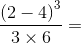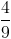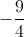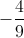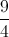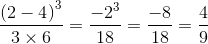# Algebra 1 : How to multiply integers

## Example Questions

← Previous 1 3 4 5 6

### Example Question #61 : Real Numbers

Evaluate 5x + 3y when x = 7 and y = 9.

66

8

–66

–62

62

62

Explanation:

Plug in 7 for x and 5 for y, giving you 5(7) + 3(9). This is equal to 35 + 27, which equals 62.

5x + 3y

5(7) + 3(9)

35 + 27 = 62

### Example Question #1 : How To Multiply Integers

A plane flies at a rate of 210mi/h. If the plane has flown for five hours, how many miles has it traveled?

42mi

1050mi

1000mi

840mi

215mi

1050mi

Explanation:

Multiply the rate of travel (210 mi/hr) by the time traveled (5 hours), and you get the total distance, 1050 miles.

distance = rate * time

distance = (210mi/hr)(5hr)

distance = 1050mi

### Example Question #2 : How To Multiply Integers

Find the volume of a cube that is five feet on each side.

10ft3

25ft3

125ft3

5ft3

15ft3

125ft3

Explanation:

To find the volume of a cube, you cube the side length, raising it to the thrid power. 5 cubed is 125.

(5ft)3 = 5ft*5ft*5ft = 125ft3

### Example Question #1 : How To Multiply Integers

What is the square root of 81?

–9

9

40.5

3

27

9

Explanation:

9 * 9 = 81, and thus 9 is the square root of 81. It is true that (–9) * (–9) = 81, but it is a convention that "the square root" refers to the positive value.  Had the question asked for "a square root" or "the square roots" of 81, –9 would be acceptable.

### Example Question #3 : How To Multiply Integers

What is (–16) * (–2)?

–8

32

8

–32

64

32

Explanation:

When multiplying two negative numbers, the negatives cancel each other out, leaving a positive solution, thus, we can think of (–16)(–2) as (16)(2), which is 32.

(–16) * (–2)

(–1)(16) * (–1)(2)

(–1)(–1) * (16)(2)

(1) * (16)(2)

16 * 2

32

### Example Question #4 : How To Multiply Integers

Solve: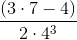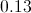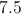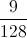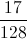Explanation: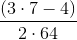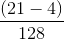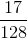### Example Question #5 : How To Multiply Integers

Ifis an integer, which of the following must be odd?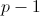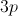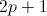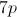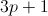Explanation:

While all of the answers could result in an odd answer, only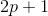guarantees an odd answer.  Since any integer multiplied by 2 is even, adding 1 will always give the next consecutive odd integer.

### Example Question #77 : Integer Operations

What is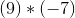?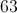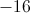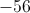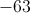Explanation:

Multiply the absolute values to get the magnitude of the answer.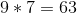Remember the following rules:

Positive * negative = negative

Positive * positive = positive

Negative * negative = positive

Since we are dealing with a positive and a negative, our answer will be negative.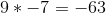### Example Question #6 : How To Multiply Integers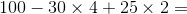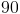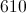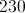Explanation: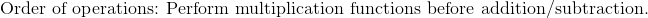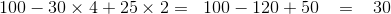### Example Question #7 : How To Multiply Integers